# Volume 20

## Pseudo-Dynamic Approach to the Numerical Solution of Nonlinear Stationary Partial Differential Equations »NBCDFPDF

Published October 8, 2018
dx.doi.org/doi:10.3888/tmj.20-8
This article presents a numerical pseudo-dynamic approach to solve a nonlinear stationary partial differential equation (PDE)with bifurcations by passing fromto a pseudo-time-dependent PDE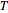. The equationis constructed so that the desired nontrivial solution ofrepresents a fixed point of. The numeric solution ofis then obtained as the solution ofat a high enough value of the

## A Wolfram Language Approach to Real Numerical Algebraic Plane Curves »NBCDFPDF

Published August 29, 2018
dx.doi.org/doi:10.3888/tmj.20-7

## Improving the Kruskal—Katona Bounds for Complete Subgraphs of a Graph »NBCDFPDF

Published July 30, 2018
dx.doi.org/doi:10.3888/tmj.20-6

An important problem in graph theory is to find the number of complete subgraphs of a given size in a graph. If the graph is very large, it is usually only possible to obtain upper bounds for these numbers based on the numbers of complete subgraphs of smaller sizes. The KruskalKatona bounds are often used for these calculations. We investigate these bounds in specific cases and study how they might be improved. Read More »

## Computational Aspects of Quaternionic Polynomials »NBCDFPDF

### Part II: Root-Finding Methods

Published July 1, 2018
dx.doi.org/doi:10.3888/tmj.20-5

This article explores the numerical mathematics and visualization capabilities of Mathematica in the framework of quaternion algebra. In this context, we discuss computational aspects of the recently introduced Newton and Weierstrass methods for finding the roots of a quaternionic polynomial. Read More »

## Computational Aspects of Quaternionic Polynomials »NBCDFPDF

### Part I: Manipulating, Evaluating and Factoring

Published May 4, 2018
dx.doi.org/doi:10.3888/tmj.20-4

This article discusses a recently developed Mathematica tool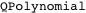a collection of functions for manipulating, evaluating and factoring quaternionic polynomials.relies on the package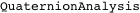, which is available for download at w3.math.uminho.pt/QuaternionAnalysis. Read More »

## The Modular Group »NBCDFPDF

### A Finitely Generated Group with Interesting Subgroups

Published March 26, 2018
dx.doi.org/doi:10.3888/tmj.20-3

The action of Möbius transformations with real coefficients preserves the hyperbolic metric in the upper half-plane model of the hyperbolic plane. The modular group is an interesting group of hyperbolic isometries generated by two Möbius transformations, namely, an order-two element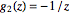and an element of infinite order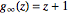. Viewing the action of the group elements on a model of the hyperbolic plane provides insight into the structure of hyperbolic 2-space. Animations provide dynamic illustrations of this action. Read More »

## Symbolic Solutions of Simultaneous First-Order PDEs in One Unknown »NBCDFPDF

Published February 26, 2018
dx.doi.org/doi:10.3888/tmj.20-2

We propose and implement an algorithm for solving an overdetermined system of partial differential equations in one unknown. Our approach relies on the BourMayer method to determine compatibility conditions via JacobiMayer brackets. We solve compatible systems recursively by imitating what one would do with pen and paper: Solve one equation, substitute its solution into the remaining equations, and iterate the process until the equations of the system are exhausted. The method we employ for assessing the consistency of the underlying system differs from the traditional use of differential Gröbner bases, yet seems more efficient and straightforward to implement. Read More »

## A Beginner’s Guide to Solving Sudoku Puzzles by Computer »NBCDFPDF

Published January 25, 2018
dx.doi.org/doi:10.3888/tmj.20-1

We simultaneously introduce effective techniques for solving Sudoku puzzles and explain how to implement them in Mathematica. The hardest puzzles require some guessing, and we include a simple backtracking technique that solves even the hardest puzzles. The programming skills required are kept at a minimum. Read More »